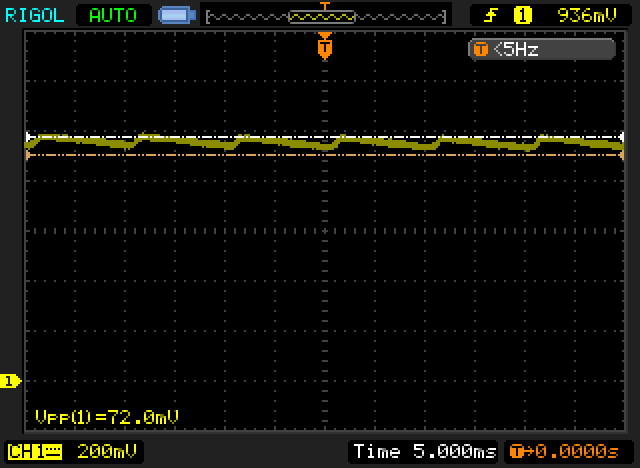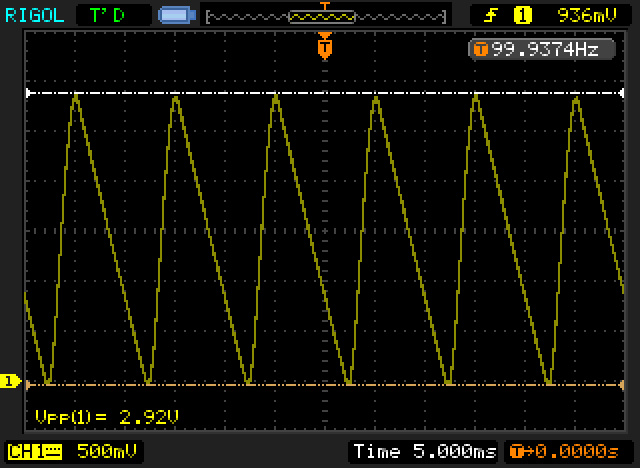# Effective Use of the Capacitance Multiplier for Voltage Regulators

This post discusses a topic I’ve shared quite a long time ago on a few other forums, I’ve decided to post it here on the blog just in case it will become unavailable on these forums at some point, as it is a fairly old post. I don’t have the original schematics anymore, so bare with the lower res images I’m copying over from my original post.

Many voltage regulators use the capacitance multiplier as a method of increasing the effective capacitance seen by a load. Some use it as a complete voltage “regulator” (although its more of a filter in that case than it is a regulator), while others use it as a low-pass-filter (LPF) for the error amplifier at the core of the regulator. The basic idea is to use a BJT transistor as a follower to amplify the capacitor current by ~hfe (small signal current gain) of the transistor, making the capacitor appear as if it was ~hfe larger in value. This simple structure is shown in Fig. 1.

R1+C2 form a LPF, which is buffered by T1, these 3 devices comprise the capacitance multiplier. This filtered voltage is used to power the error amplifier which drives the pass transistor and takes a sample of the output voltage by the R2/R3 voltage divider. The reference voltage isn’t shown in this diagram for simplicity. This circuit is very simple to understand, and is a fairly close representation of many voltage regulator designs. C1 is the bulk filter capacitor, and can be preceded by a rectifier, or any other source of power. Under light load condition there is nothing interesting in this circuit, and it behaves as expected. However, as soon as we start sourcing appreciable current at the output by the load, the voltage over the bulk capacitor (C1) will fluctuate considerably. If it has sufficient ripple, transistor T1 can no longer be assumed as operating in the forward active region, and can actually start conducting from C2 to C1. This will obviously prevent the capacitance multiplier from operating properly, and will translate to ripple on the supply of the error amplifier, and therefore the output voltage.

In Fig. 2 we can see VCE(volts) and IC(mA’s) of T1 for this circuit with a 3300uF C1, 1K+100uF (R1/C2) LPF, and a load of 1A (the results are from pSpice with the error-amp biased at ~10mA). As can be seen above, these conditions are sufficient to make the transistor conduct in the opposite direction at the end of each cycle, which means the capacitance multiplier is no longer operating properly. This simulation was carried out with a full wave rectifier and a 50Hz sin source.

However, the capacitance multiplier is still a very simple circuit that we would like to exploit for its effective filtering. Lucky for us, this issue can be solved quite easily by adding 2 cheap component, as can be seen in Fig. 3 below. Here we have added D1 and C4. The combination of these 2 devices allows us to isolate the capacitance multiplier from the large ripple present over C1. This is basically a peak-detector circuit that we use as the supply for the capacitance multiplier. When the voltage over C1 is high enough, D1 will conduct and C4 will be charged. When the voltage over C1 drops, D1 is not conducting, and C4 is used as the charge reservoir supplying power to the capacitance multiplier. Since C4 need only supply the error amplifier (and in some cases the reference), it can be fairly small while having limited ripple.

D1 can be any diode you’d like, but using a low voltage drop diode will reduce the dropout voltage of the regulator, so it is recommended. C4 can be calculated fairly easily. Depending on the simplification you make you will get a different value, but they are all fairly small and inexpensive. R1/C2 forms a LPF so lets assume the voltage at the base of T1 is the average of the voltage at its collector. Using this assumption with the fact a BJT can operate with VCE as low as ~0.2V (and we know VBE=~0.7), we can easily find that a ripple of up to 1Vpk-pk over C4 is tolerable.  We can now use the charge equation of a capacitor and rearrange this slightly and find:

$C=I}{\left(2*Freq*∆V\right)}$

where I is the load current, Freq is the mains frequency, the factor 2 assumes a full-wave rectifier (which effectively doubles the frequency), and the final term is the voltage drop we allow. In the example here I will use 10mA, 50Hz, and 1V. This results in ~100uF. We can double that to 200uF (220uF would be a practical value), to keep the transistor operating close to its nominal point and reduce ripple further. Fig. 4 shows the same waveforms as Fig. 2, but this time with the added components.

we can see a significant improvement in both reduced voltage ripple over the transistor, as well as a well behaved transistor current that meets our expectations from the small-signal analysis of such a circuit.

To estimate practical number, I’ve used a single-rail power-supply that I’ve owned at the time and used to power one of the headphone amplifiers. It used a structure that is closely matched by the simplified diagram of Fig. 1. The output voltage was set to 24V (23.3V to be exact), and it was loaded with a load of ~1A (15ohm + 8ohm resistors in series). The component values such as bulk capacitor C1 were similar to these used in simulation. Integrated noise measurements were made with the LNMP from Tangent and an Agilent U1253A, and waveforms were captured with the Rigol  DS1052E I’ve owned at the time.

Results pre-modification:
Here’s VCE of Q1 under these conditions:
No load:Fig. 5. VCE Ripple with 0A Load

1A load:Fig. 6. VCE Ripple with 1A Load

As can be observed in Fig. 6, VCE of T1 fluctuates significantly, and drops down to 0V, which means the capacitance multiplier isn’t operating as expected. This can obviously be even more severe with higher load currents.

Results post-modification:
I’ve used UF1004 diode and 330uF capacitor for the mod, as these are  the closest values I’ve had at the moment of testing.